# Fitting LISREL Models by the LISMOD Modeling Language

The model described in the section Career Aspiration: Analysis 3 provides a good example of the LISREL model. In PROC CALIS, the LISREL model specifications are supported by a matrix-based language called LISMOD (LISREL model). In this section, the path diagram in Figure 17.44 is specified by the LISMOD modeling language of PROC CALIS. See the section Career Aspiration: Analysis 3 for detailed descriptions of the model.

In order to understand the LISMOD modeling language of PROC CALIS, some basic understanding of the LISREL model is necessary. In a LISREL model, variables are classified into four distinct classes:

•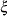is a vector of exogenous (independent) latent variables in the model. They are specified in XI= variable list in the LISMOD statement.

•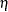is a vector of endogenous (dependent) latent variables in the model. They are specified in ETA= variable list in the LISMOD statement.

•is a vector of observed indicator variables forin the model. They are specified in XVAR= variable list in the LISMOD statement.

•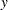is a vector of observed indicator variables forin the model. They are specified in YVAR= variable list in the LISMOD statement.

For detailed descriptions of the LISMOD modeling language, see the LISMOD statement and the section The LISMOD Model and Submodels. To successfully set up a LISMOD model in PROC CALIS, you first need to recognize these classes of variables in your model. For the path diagram in Figure 17.44, it is not difficult to see the following:

•is the vector of the intelligence and environmental factors: f_rpa, f_riq, f_rses, f_fses, f_fiq, and f_fpa. These variables are exogenous because no single-headed arrows point to them.

•is the vector of the ambition factors: R_Amb, and F_Amb. They are endogenous because each of them has at least one single-headed arrow pointing to it.

•is the vector of the observed indicator variables for the intelligence and environmental factors. These indicators are rpa, riq, rses, fses, fiq, and fpa.

•is the vector of observed indicator variables for the ambition factors. These indicators are rea, roa, foa, and fea.

In LISMOD, you do not need to define error terms explicitly as latent variables. The parameters in LISMOD are defined as entries in various model matrices. The following statements specify the LISMOD model for the diagram in Figure 17.44:

```proc calis data=aspire nobs=329;
lismod
xi   = f_rpa f_riq f_rses f_fses f_fiq f_fpa,
eta  = R_Amb F_Amb,
xvar = rpa riq rses fses fiq fpa,
yvar = rea roa foa fea;

/* measurement model for aspiration */
matrix _lambday_ [1,1], [2,1] = 1.0, [3,2] = 1.0, [4,2];
matrix _thetay_  [4,1], [3,2];

/* measurement model for intelligence and environment */
matrix _lambdax_ [1,1] = 0.837 0.894 0.949 0.949 0.894 0.837;

/* structural model of influences */
matrix _beta_ [2,1],[1,2];
matrix _gamma_ [1,1 to 4], [2,3 to 6];

/* Covariances among Eta-variables */
matrix _psi_ [2,1];

/* Fixed variances for Xi-variables */
matrix _phi_ [1,1] = 6 * 1.0;
run;
```

The LISMOD statement invokes the LISMOD modeling language of PROC CALIS. In the LISMOD statement, you list the four classes of variables in the model in the XI=, ETA=, XVAR=, and YVAR= variable lists, respectively. After you define the four classes of variables, you use several MATRIX statements to specify the model matrices and the parameters in the model.

Basically, there are three model components in the LISMOD specification: two measurement models and one structural model. The first measurement model specifies the functional relationships between observed variables(YVAR= variables) and the endogenous (dependent) latent factors(ETA= variables). The second measurement model specifies the functional relationships between observed variablesand (XVAR= variables) and the exogenous (independent) latent factors(XI= variables). The structural model specifies the relationships between the endogenous and exogenous latent variablesand. To facilitate the discussion of these model components and the corresponding LISMOD model specification, some initial model output from PROC CALIS are shown.

### The Measurement Model forThe first component of the LISMOD specification is the measurement model for, as shown in the following equation: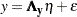In the context of covariance structure analysis, without loss of generality, it is assumed thatandare centered so that there is no intercept term in the equation. This equation essentially states thatis a function of the true scores vectorplus the error term, which is independent of. The model matrices involved in this measurement model are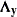(effects ofon) and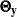, which is the covariance matrix of.

For the career aspiration data, you specify the following two MATRIX statements for this measurement model:

```   matrix _lambday_ [1,1], [2,1] = 1.0, [3,2] = 1.0, [4,2];
matrix _thetay_  [4,1], [3,2];
```

The first matrix statement is for matrix. You specify four parameters in this matrix. The [1,1] and [4,2] elements are free parameters, and the [2,1] and [3,2] elements have fixed values of 1. You do not specify other elements in this matrix. By default, unspecified elements in thematrix are fixed zeros. You can check your initial model specification of this matrix, as shown in the Figure 17.47.

Figure 17.47 Career Aspiration Analysis 3: Initial Measurement Model for y
Initial _LAMBDAY_ Matrix
R_Amb F_Amb
rea
 . [_Parm01]
 0
roa
 1.0000
 0
foa
 0
 1.0000
fea
 0
 . [_Parm02]

Initial _THETAY_ Matrix
rea roa foa fea
rea
 0
 0
 . [_Parm13]
roa
 0
 . [_Parm14]
 0
foa
 0
 . [_Parm14]
 0
fea
 . [_Parm13]
 0
 0

In Figure 17.47, the initial _LAMBDAY_ matrix is a 4
2 matrix. The _LAMBDAY_ matrix contains information about the relationships between the row indicator variables(YVAR= variables) and the column factors(ETA= variables). As specified in the MATRIX statement for _LAMBDAY_, the [1,1] and [4,2] are free parameters named _Parm01 and _Parm02, respectively. These parameter names are generated by PROC CALIS. Fixed values 1.0 appear in the [2,1] and [3,2] elements. These fixed values are used to identify the scales of the latent variables R_Amb and F_Amb.

The _THETAY_ matrix in Figure 17.47 is the covariance matrix among the error terms for the-variables (YVAR= variables). This is a 44 matrix for the four measured indicators. As specified in the MATRIX statement for _THETAY_, the [4,1] and [3,2] elements are free parameters named _Parm13 and _Parm14, respectively. Because _THETAY_ is a symmetric matrix, elements [1,4] and [2,3] are also implicitly specified as parameters in this model matrix.

As shown in Figure 17.47, PROC CALIS adds four default free parameters to the _THETAY_ matrix. On the diagonal of the _THETAY_ matrix, parameters _Add07, _Add08, _Add09, and _Add10 are added as default free parameters by PROC CALIS automatically. In general, error variances are default free parameters in PROC CALIS. You do not have to specify them but you can specify them if you want to, especially when you need to set fixed values or other constraints on them.

### The Measurement Model forThe second component of the LISMOD specification is the measurement model for, as shown in the following equation: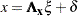The measurement model foris similar to that for. Assuming thatandare centered, this equation states thatis a function of the true scores vectorplus the error term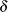, which is independent of. The model matrices involved in this measurement model are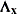(effects ofon) and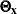, which is the covariance matrix of.

For the career aspiration data, you specify the following MATRIX statement for this measurement model:

```   matrix _lambdax_ [1,1] = 0.837 0.894 0.949 0.949 0.894 0.837;
```

Figure 17.48 shows the output related to the specification of the measurement model for.

Figure 17.48 Career Aspiration Analysis 3: Initial Measurement Model for x
Initial _LAMBDAX_ Matrix
f_rpa f_riq f_rses f_fses f_fiq f_fpa
rpa 0.8370 0 0 0 0 0
riq 0 0.8940 0 0 0 0
rses 0 0 0.9490 0 0 0
fses 0 0 0 0.9490 0 0
fiq 0 0 0 0 0.8940 0
fpa 0 0 0 0 0 0.8370

Initial _THETAX_ Matrix
rpa riq rses fses fiq fpa
rpa
 0
 0
 0
 0
 0
riq
 0
 0
 0
 0
 0
rses
 0
 0
 0
 0
 0
fses
 0
 0
 0
 0
 0
fiq
 0
 0
 0
 0
 0
fpa
 0
 0
 0
 0
 0

In Figure 17.48, the initial _LAMBDAX_ matrix is a 66 matrix. The _LAMBDAX_ matrix contains information about the relationships between the row indicator variables(XVAR= variables) and the column factors(XI= variables). As specified in the MATRIX statement for _LAMBDAX_, the diagonal elements are filled with the fixed values provided. The [1,1] specification in the MATRIX statement for _LAMBDAX_ provides the starting element for the subsequent parameter list to fill in. In this case, the list contains six fixed values, and PROC CALIS proceeds from [1,1] to [2,2], [3,3] and so on until the entire list of parameters is consumed. This kind of notation is a shortcut of the following equivalent specification:

```   matrix _lambdax_ [1,1]=0.837, [2,2]=0.894, [3,3]=0.949,
[4,4]=0.949, [5,5]=0.894, [6,6]=0.837;
```

PROC CALIS provides many different kinds of shortcuts in specifying matrix elements. See the MATRIX statement of Chapter 26, The CALIS Procedure, for details.

At the bottom of Figure 17.48, the initial _THETAX_ matrix is shown. Even though you did not specify any elements of this matrix in any MATRIX statements, the diagonal elements of this matrix are set as default parameters by PROC CALIS. Default parameters added by PROC CALIS are all denoted by names with the prefix '_Add'.

### The Structural Model

The last component of the LISMOD specification is the structural model that describes the relationship betweenand, as shown in the following equation: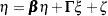In this equation,is endogenous (dependent) andis exogenous (independent). Variables incan have effects among themselves. Their effects are specified in thematrix. The effects ofonare specified in the
matrix. Finally, the error term for the structural relationships is denoted by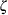, which is independent of.

There are four model matrices assumed in the structural model.andare matrices for the effects of variables. In addition, matrix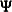denotes the covariance matrix for the error term, and matrix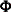denotes the covariance matrix of.

For the career aspiration data, you use the following MATRIX statements for the structural model:

```   matrix _beta_ [2,1],[1,2];
matrix _gamma_ [1,1 to 4], [2,3 to 6];
matrix _psi_ [2,1];
matrix _phi_ [1,1] = 6 * 1.0;
```

In Figure 17.49, initial _BETA_ and _GAMMA_ matrices are shown.

Figure 17.49 Career Aspiration Analysis 3: Initial Structural Equations
Initial _BETA_ Matrix
R_Amb F_Amb
R_Amb
 0
 . [_Parm12]
F_Amb
 . [_Parm11]
 0

Initial _GAMMA_ Matrix
f_rpa f_riq f_rses f_fses f_fiq f_fpa
R_Amb
 . [_Parm03]
 . [_Parm04]
 . [_Parm05]
 . [_Parm06]
 0
 0
F_Amb
 0
 0
 . [_Parm07]
 . [_Parm08]
 . [_Parm09]
 . [_Parm10]

In Figure 17.49, the _BETA_ matrix contains information about the relationships among the-variables (ETA= variables). Both the row and column variables of the _BETA_ matrix refer to the list of-variables. The row variables receive effects from the column variables. You specify two parameters in the _BETA_ matrix: element [2,1] is the effect of R_Amb on F_Amb, and element [2,1] is the effect of F_Amb on R_Amb. Other effects are fixed zeros in this matrix.

The _GAMMA_ matrix contains information about the relationships between the-variables (ETA= variables) and the-variables (XI= variables). The row variables are the-variables, which receive effects from the column-variables. You specify eight free parameters in this matrix. These eight parameters represent the eight path coefficients from(the intelligence and environment factors) to thevariables (the ambition factors), as shown in the path diagram in Figure 17.44. A shortcut in the MATRIX statement syntax for the _GAMMA_ matrix has been used. That is, [1, 1 to 4] means the [1,1], [1,2], [1,3], and [1,4] elements, and [2, 3 to 6] means the [2,3], [2,4], [2,5], and [2,6] elements. All these elements are free parameters in the model and free parameter names are generated for these elements.

Figure 17.50 shows the initial _PSI_ and _PHI_ matrices.

Figure 17.50 Career Aspiration Analysis 3: Initial Variances and Covariances
Initial _PSI_ Matrix
R_Amb F_Amb
R_Amb
 . [_Parm15]
F_Amb
 . [_Parm15]

Initial _PHI_ Matrix
f_rpa f_riq f_rses f_fses f_fiq f_fpa
f_rpa
 1.0000
f_riq
 1.0000
f_rses
 1.0000
f_fses
 1.0000
f_fiq
 1.0000
f_fpa
 1.0000

The _PSI_ matrix contains information about the covariances of error terms for the-variables, which are endogenous in the structural model. There are two-variables in the model—the two ambition factors R_Amb and F_Amb. You specify the [2,1] element as a free parameter in the MATRIX statement for _PSI_. This means that the error covariance between R_Amb and F_Amb is a free parameter to estimate in the model. In Figure 17.50, both [2,1] and [1,2] elements are named as _Parm15 because _PSI_ is a symmetric matrix. Again, the diagonal elements of this covariance matrix, which are for the error variances of the ambition factors, are default free parameters in PROC CALIS. These parameters are named with the prefix _Add.

Finally, the _PHI_ matrix contains information about the covariances among the exogenous latent factors in the structural model. For the _PHI_ matrix, you fix all the diagonal elements to 1 in the MATRIX statement for _PHI_. This makes the latent variable scales identified. These fixed values are echoed in the output of the initial _PHI_ matrix shown in Figure 17.50. In addition, all covariances among latent exogenous variables are set to be free parameters by default.

### Fit Summary of the LISMOD Model for Career Aspiration Analysis 3

Figure 17.51 shows the fit summary of the LISMOD model. All these fit index values match those from using the PATH model specification of the same model, as shown in Figure 17.45. Therefore, you are confident that the current LISMOD model specification is equivalent to the PATH model specification shown in the section Career Aspiration: Analysis 3.

Figure 17.51 Career Aspiration Analysis 3: Fit Summary of the LISMOD Model
Fit Summary
Chi-Square 12.0132
Chi-Square DF 13
Pr > Chi-Square 0.5266
Standardized RMSR (SRMSR) 0.0149
RMSEA Estimate 0.0000
Akaike Information Criterion 96.0132
Schwarz Bayesian Criterion 255.4476
Bentler Comparative Fit Index 1.0000

Estimation results are shown in Figure 17.52, Figure 17.53, and Figure 17.54, respectively for the measurement model for, measurement model for, and the structural model. These are the same estimation results as those from the equivalent PATH model specification in Figure 17.46. However, estimates in the LISMOD model are now arranged in the matrix form, with standard error estimates andvalues shown.

Figure 17.52 Career Aspiration Analysis 3: Estimation of Measurement Model for y
_LAMBDAY_ Matrix: Estimate/StdErr/t-value
R_Amb F_Amb
rea
 1.0840 0.0942 11.5105 [_Parm01]
 0
roa
 1.0000
 0
foa
 0
 1.0000
fea
 0
 1.1163 0.0863 12.9394 [_Parm02]

_THETAY_ Matrix: Estimate/StdErr/t-value
rea roa foa fea
rea
 0
 0
 0.0231 0.0314 0.7355 [_Parm13]
roa
 0
 0.1121 0.0326 3.4399 [_Parm14]
 0
foa
 0
 0.1121 0.0326 3.4399 [_Parm14]
 0
fea
 0.0231 0.0314 0.7355 [_Parm13]
 0
 0

Figure 17.53 Career Aspiration Analysis 3: Estimation of Measurement Model for x
_LAMBDAX_ Matrix: Estimate/StdErr/t-value
f_rpa f_riq f_rses f_fses f_fiq f_fpa
rpa
 0.8370
 0
 0
 0
 0
 0
riq
 0
 0.8940
 0
 0
 0
 0
rses
 0
 0
 0.9490
 0
 0
 0
fses
 0
 0
 0
 0.9490
 0
 0
fiq
 0
 0
 0
 0
 0.8940
 0
fpa
 0
 0
 0
 0
 0
 0.8370

_THETAX_ Matrix: Estimate/StdErr/t-value
rpa riq rses fses fiq fpa
rpa
 0
 0
 0
 0
 0
riq
 0
 0
 0
 0
 0
rses
 0
 0
 0
 0
 0
fses
 0
 0
 0
 0
 0
fiq
 0
 0
 0
 0
 0
fpa
 0
 0
 0
 0
 0

Figure 17.54 Career Aspiration Analysis 3: Estimation of Structural Model
_BETA_ Matrix: Estimate/StdErr/t-value
R_Amb F_Amb
R_Amb
 0
 0.1190 0.1140 1.0441 [_Parm12]
F_Amb
 0.1302 0.1207 1.0791 [_Parm11]
 0

_GAMMA_ Matrix: Estimate/StdErr/t-value
f_rpa f_riq f_rses f_fses f_fiq f_fpa
R_Amb
 0.1837 0.0504 3.6420 [_Parm03]
 0.2800 0.0614 4.5618 [_Parm04]
 0.2262 0.0522 4.3300 [_Parm05]
 0.0870 0.0548 1.5883 [_Parm06]
 0
 0
F_Amb
 0
 0
 0.0633 0.0522 1.2124 [_Parm07]
 0.2154 0.0512 4.2060 [_Parm08]
 0.3539 0.0674 5.2497 [_Parm09]
 0.1688 0.0493 3.4205 [_Parm10]

_PSI_ Matrix: Estimate/StdErr/t-value
R_Amb F_Amb
R_Amb
 -0.009355 0.0501 -0.1867 [_Parm15]
F_Amb
 -0.009355 0.0501 -0.1867 [_Parm15]

_PHI_ Matrix: Estimate/StdErr/t-value
f_rpa f_riq f_rses f_fses f_fiq f_fpa
f_rpa
 1.0000
f_riq
 1.0000
f_rses
 1.0000
f_fses
 1.0000
f_fiq